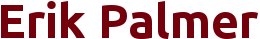# Courses Taught

## University of South Carolina, Columbia

• ### Math 111 - Basic College Mathematics

Fall 2017 - Currently Teaching
• Basic college algebra; linear and quadratic equations, inequalities, functions and graphs of functions, exponential and logarithm functions, systems of equations.

• ### Math 242 - Elementary Differential Equations

Fall 2016
• Ordinary differential equations of first order, higher order linear equations, Laplace transform methods, series methods; numerical solutions of differential equations. Applications to physical sciences and engineering.

• ### Math 115 - Precaclulus

Fall 2015
• Topics in algebra and trigonometry: Subsets of the real line, absolute value; polynomial, rational, inverse, logarithmic, and exponential functions; circular functions; analytic trigonometry.

• ### Math 142 - Calculus 2

Teaching Assistant: Spring 2014, Fall 2014
• Methods of integrations, sequences and series, approximations. One hour computer lab.

• ### Math 111I -Intensive Basic College Mathematics

Fall 2013
• Basic college algebra; linear and quadratic equations, inequalities, functions and graphs of functions, exponential and logarithm functions, systems of equations.

## California State University, East Bay

• ### Math 800 - Introduction to Algebra:

Winter 2011 (801), Fall 2012
• Fractions, signed numbers, percentages, introduction to geometry, simplifying algebraic expressions, solving linear equations, straight lines.

• ### Math 900 - Elementary Algebra:

Fall 2011, Winter 2013
• A one quarter course in elementary algebra.

• ### Math 950 - Intermediate Algebra:

Fall 2012, Winter 2012, Winter 2013, Spring 2013 (2 sections)
• Operations with algebraic expressions, exponents and radicals; linear and quadratic functions and their graphs; elementary conic sections; word problems.